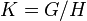# Number of conjugacy classes in a quotient is less than or equal to number of conjugacy classes of group

## Statement

Suppose$G$ is a group,$H$ is a normal subgroup, and$K = G/H$ is the Quotient group (?). Then, the Number of conjugacy classes (?) of$K$ is less than or equal to the number of conjugacy classes of$G$.

When$G$ is a finite group, both numbers are finite and this can be thought of as a comparison of finite numbers. When$G$ is infinite, one or both numbers could potentially be infinite and the statement can be viewed in terms of comparisons of infinite cardinals.

Finally, the number of conjugacy classes in$K$ is strictly less than the number in$G$ if$G$ is finite and$H$ is nontrivial.

## Proof

Given: A group$G$, a normal subgroup$H$, quotient$K = G/H$, with quotient map$q:G \to K$. Let$C(G)$ and$C(K)$ be the sets of conjugacy classes in$G$ and$K$ respectively.

To prove:$|C(K)| \le |C(G)|$, and if$G$ is finite and$H$ nontrivial, then$|C(K)| < |C(G)|$.

Proof:

Step no. Assertion/construction Facts used Given data used Previous steps used Explanation
1 The quotient map from$G$ to$K$ sends conjugate elements to conjugate elements, hence induces a set map$q_1:C(G) \to C(K)$
2 The set map$q_1$ from Step (1) is surjective.
3 If$H$ is nontrivial,$q_1$ is not injective.
4$|C(K)| \le |C(G)|$ and inequality is strict if$G$ is finite and$H$ is nontrivial.